# Leetcode 算法面试冲刺 实战 六（数组与循环）（十三）2133-Jammy

, , ,

# 768 · 杨辉三角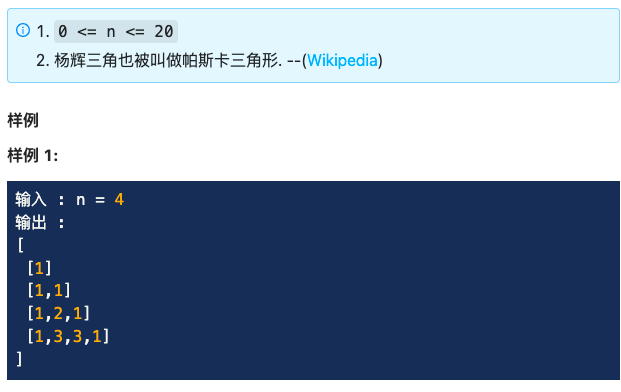def calcYangHuisTriangle(self, n):
if n <= 0: return []
elif n == 1: return []
elif n == 2: return [, [1, 1]]

li = [, [1, 1]]
for i in range(2, n):
temp = 
for j in range(1, i):
temp.append(li[i-1][j-1] + li[i-1][j])
temp.append(1)
li.append(temp)
return lidef calcYangHuisTriangle(self, n):
res = [ * i for i in range(1,n+1)]
for row in range(2,n):
for col in range (1,row):
res[row][col] = res[row-1][col-1] + res[row-1][col]
return res


# 767 · 翻转数组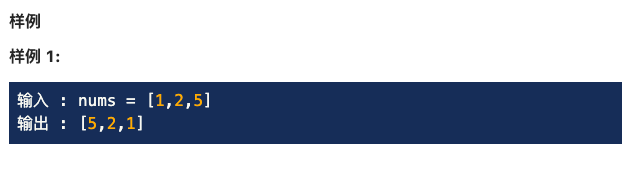def reverseArray(self, nums):
left, right = 0, len(nums) - 1
while left <= right:
nums[left], nums[right] = nums[right], nums[left]
left += 1
right -= 1
return numsdef reverseArray(self, nums):
n = len(nums)
for i in range(n//2):
nums[i], nums[n-i-1] = nums[n-i-1], nums[i]
return nums


# 539 · 移动零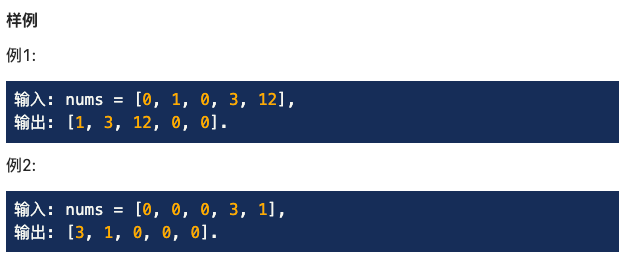def moveZeroes(self, nums):
if not nums: return
left, right = 0, len(nums) - 1
while left <= right:
while (left < right) and (nums[left] != 0): left += 1
while (left < right) and (nums[right] == 0): right -= 1
if left == right: break
nums[left], nums[right] = nums[right], nums[left]
return nums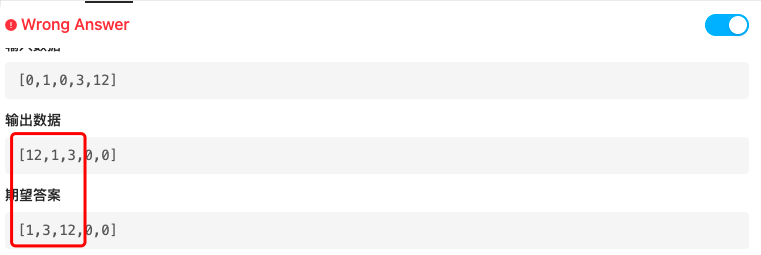def moveZeroes(self, nums):
zero, nonzero = 0, 0
n = len(nums)
while nonzero < n - 1 and zero < n - 1:
while nonzero < n - 1 and nums[nonzero] == 0: nonzero += 1
while zero < n - 1 and nums[zero] != 0: zero += 1
if zero < nonzero:
nums[zero], nums[nonzero] = nums[nonzero], nums[zero]
elif nonzero == n - 1 or zero == n - 1: break
elif zero > nonzero: nonzero += 1def moveZeroes(self, nums):
left, right = 0, 0
while right < len(nums):
if nums[right] != 0:
nums[left], nums[right] = nums[right], nums[left]
left += 1
right += 1


# 479 · 数组第二大数import heapq
class Solution:
"""
@param nums: An integer array
@return: The second max number in the array.
"""

def secondMax(self, nums):
que = []
heapq.heapify(que)
for num in nums:
heapq.heappush(que, num)
if len(que) > 2:
heapq.heappop(que)
return que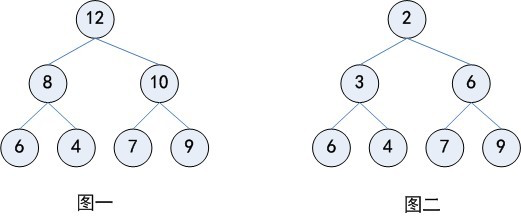class KthLargest(object):

def __init__(self, k, nums):
"""
:type k: int
:type nums: List[int]
"""
self.k = k
self.que = nums
heapq.heapify(self.que)

"""
:type val: int
:rtype: int
"""
heapq.heappush(self.que, val)
while len(self.que) > self.k:
heapq.heappop(self.que)
return self.que

# Your KthLargest object will be instantiated and called as such:
# obj = KthLargest(k, nums)


def secondMax(self, nums):
maxValue = max(nums, nums)
secValue = min(nums, nums)

for i in xrange(2, len(nums)):
if nums[i] > maxValue:
secValue = maxValue
maxValue = nums[i]
elif nums[i] > secValue:
secValue = nums[i]

return secValue


# 235 · 分解质因数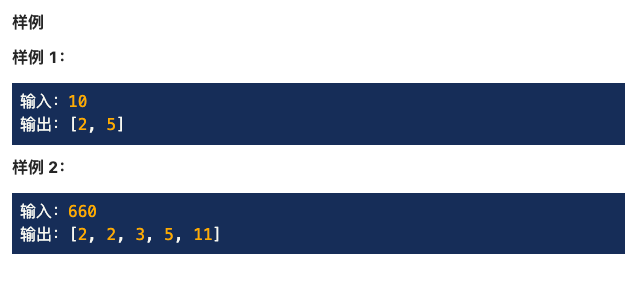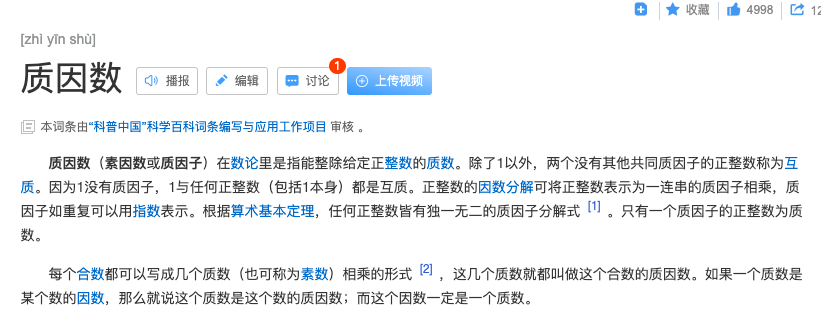class Solution:
"""
@param num: An integer
@return: an integer array
"""
def primeFactorization(self, num):
li = []
return self.derive(num, li)

def derive(self, num, li):
for n in range(2, num):
if num % n == 0:
li.append(n)
return self.derive(num // n, li)
li.append(num)
return li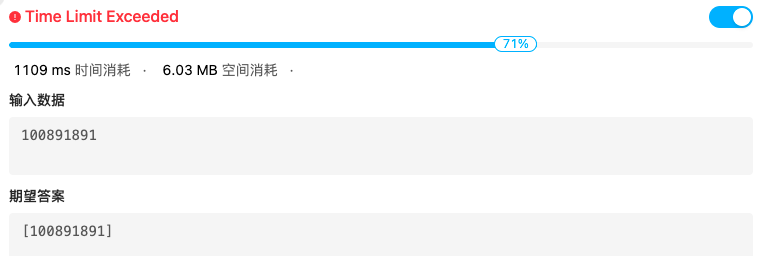up一般设定为sqrt(num)，因为一个数大于其根号的质因数最多只有一个，那么遍历其根号内的数可以将时间复杂度减小至根号n，若遍历完prime后该数不为1，则其值为最后一个质因数

def primeFactorization(self, num):
up = int(num**0.5 + 1)
ans = []
for i in range(2,up):
while num % i == 0:
num /= i
ans += [i]
# 若最后剩余数不为1，则为最后一个质因数
if num != 1:
ans += [int(num)]
return ans


### 评论 抢沙发### 觉得文章有用就打赏一下文章作者

#### 支付宝扫一扫打赏#### 微信扫一扫打赏Vieu3.3主题Q Q 登 录